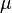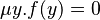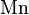# User:IssaRice/Computability and logic/Least search operator

The least search operator, minimzation operator, or μ-operator is used in computability theory to define new functions.

The least search operator allows us to move from primitive recursive functions to general recursive (total or partial) functions.

## Definition

• note: the least search operator may run into an "infinite loop". This means that partial recursive functions are not in general total.

## Notation

Most texts seem to write the least search operator was$\mu$ as in$\mu y[f(y) = 0]$ or maybe$\mu y.f(y) = 0$ (like the$\lambda$ operator for defining anonymous functions).

Boolos/Burgess/Jeffrey use$\mathrm{Mn}$ (for "minimization").

## Intuition

bounded search vs unbounded search; bounded for-loop vs while-loop.